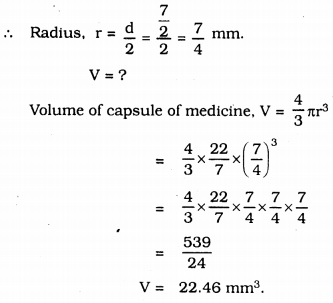# KSEEB Solutions for Class 9 Maths Chapter 13 Surface Areas and Volumes Ex 13.8

## Karnataka Board Class 9 Maths Chapter 13 Surface Areas and Volumes Ex 13.8

(Assume π = $$\frac{22}{7}$$, unless stated otherwise)

Question 1.
Find the volume of a sphere whose radius is
(i) 7 cm
(ii) 0.63 m
Solution:
(i) r = 7 cm, V = ?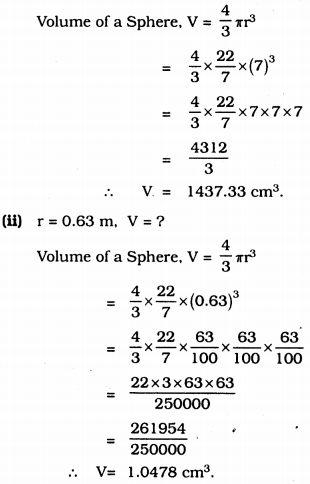Question 2.
Find the amount of water displaced by a solid spherical ball of diameter
(i) 28 cm.
(ii) 0.21 m.
Solution:
(i) Diameter of solid spherical ball, diameter, d = 28 cm,
∴ radius, r = $$\frac{28}{2}$$ = 14 cm.
Volume of solid spherical ball, V = $$\frac{4}{3}$$ πr3.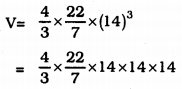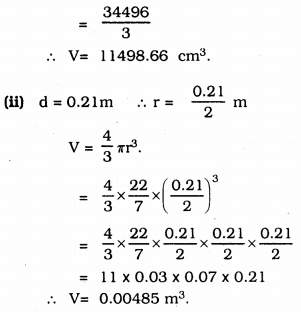Amount of water displaced by the ball = 0.00485 m3.

Question 3.
The diameter of a metallic ball is 4.2 cm. What is the mass of the ball, if the density of the metal is 8.9 g per cm3 ?
Solution:
Diameter of metallic ball, d = 4.2 cm. density = 8.9 gm/cm3
mass, m = ? d = 4.2 cm.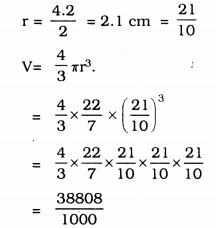∴ V = 38.808 cm3.
∴ Mass = Density × Volume
= 8.9 × 38.808
= 345.40 gm.

Question 4.
The diameter of the moon is approximately one-fourth of the diameter of the earth. What fraction of the volume of the earth is the volume of the moon?
Solution:
Let the diameter of earth be ‘d’ unit.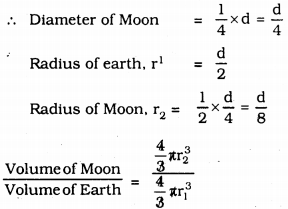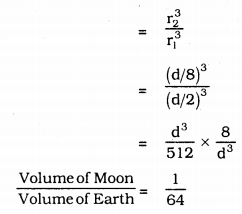∴ Volume of Moon = $$\frac{1}{64}$$ × Volume of Earth

Question 5.
How many litres of milk can a hemispherical bowl of diameter 10.5 cm hold?
Solution:
Diameter of a hemispherical bowl, d = 10.5 cm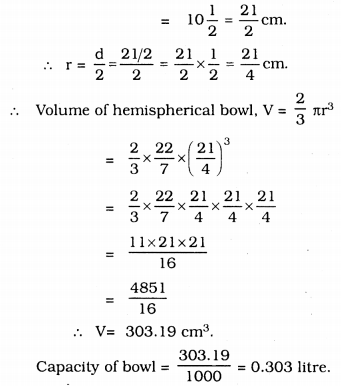Question 6.
A hemispherical tank is made up of an iron sheet 1 cm thick. If the inner radius is 1 m, then find the volume of the iron used to make the tank.
Solution:
In a hemispherical tank,
Inner radius, r1 = 1 m
Outer radius, r2 = 1 + 0.01 = 1.01 m (∵ 1 cm = 0.01)
∴ Volume of hemispherical tank, V
= Volume of outer diameter – Volume of inner diameter.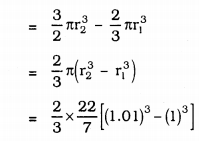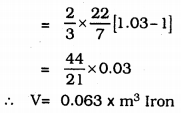Question 7.
Find the volume of sphere whose surface area is 154 cm2.
Solution:
Surface area of sphere, 4πr2 =154 cm2.
Volume of Sphere, V = ?
A = 4πr2 = 154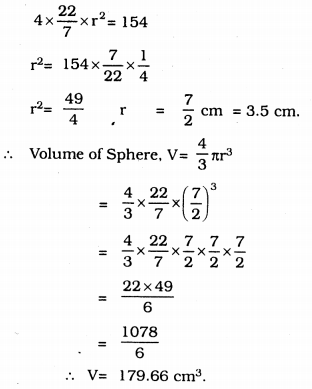Question 8.
A dome of a building is in the form of a hemisphere. From inside, it was white-washed at the cost of Rs. 498.96. If the cost of white-washing is Rs. 2.00 per square metre, find the
(i) inside surface area of the dome,
(ii) volume of the air inside the dome.
Solution:
(i) Cost of white-washing dome is Rs. 498.96
Cost of white-washing is Rs. 2 per sq. metre.
∴ Surface Area = $$\frac{498.96}{2}$$ = 249.48 m2.

(ii) Surface Area of hemisphere, V = 2πr2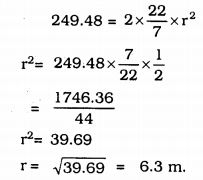∴ Inner volume of hemisphere dome, V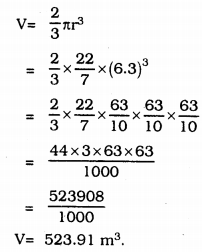Question 9.
Twenty seven solid iron spheres, each of radius r and surface area S are melted to form a sphere with surface area S1. Find the
(i) radius r of the new sphere,
(ii) ratio of S and S1
Solution:
(i) Volume of 1 sphere, V = $$\frac{4}{3}$$πr3
Volume of 27 solid sphere
= 27 × $$\frac{4}{3}$$πr3
Let r1is the radius of the new sphere.
Volume of new sphere = Volume of 27 solid sphere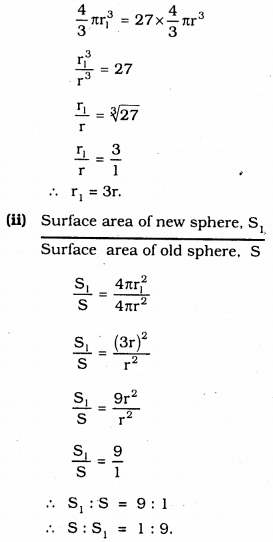Question 10.
A capsule of medicine is in the shape of a sphere of diameter 3.5 mm. How much medicine (in mm3) is needed to fill this capsule?
Solution:
Diameter of capsule of medicine, d
d = 3.5 mm = $$\frac{7}{2}$$ mm.